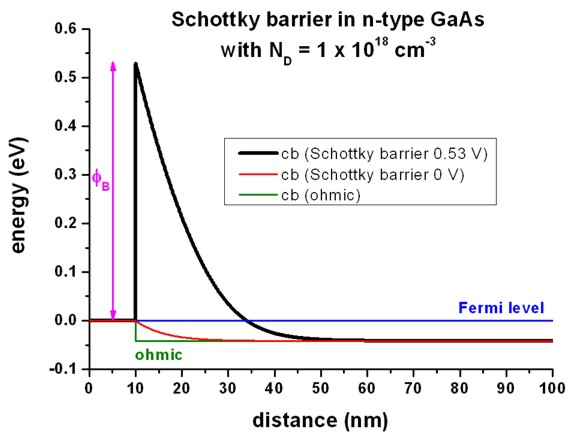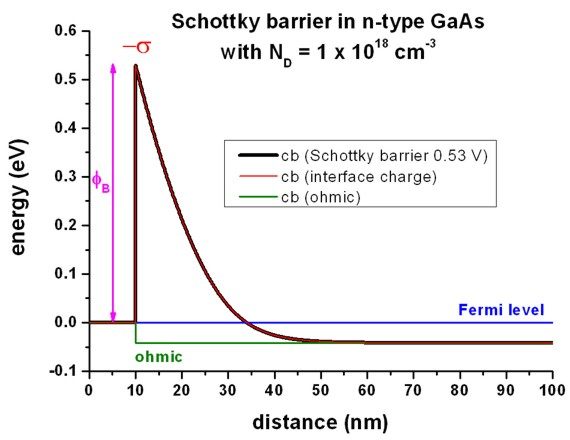nextnano.com  GUI: nextnanomat  Tool: nextnano++  Tool: nextnano³  Tool: nextnano.NEGF  Download | Search | Copyright | News | Publications  * password protected nextnano³ software1D Schottky barrier

# nextnano3 - Tutorial

## Schottky barrier

Author: Stefan Birner

```-> 1DSchottky_barrier_GaAs.in                         - ```input file for the nextnano3 and nextnano++ software (1D simulation)``` -> 2DSchottky_barrier_GaAs.in                         - ```input file for the nextnano3 and nextnano++ software (2D simulation)``` -> 1DSchottky_barrier_GaAs_ohmic.in                   - ```input file for the nextnano3 and nextnano++ software``` -> 1DSchottky_barrier_GaAs_SchottkyBarrier0V.in       - ```input file for the nextnano3 and nextnano++ software``` -> 1DSchottky_barrier_GaAs_surface_density.in         - ```input file for the nextnano3 and nextnano++ software``` -> 1DSchottky_barrier_GaAs_surface_states_acceptor.in - ```input file for the nextnano3 and nextnano++ software

`==> ` Download these input files
`    `If you don't have a password yet, you have to first sign up for a free evaluation license in order to download these input files.

## Schottky barrier

```-> 1DSchottky_barrier_GaAs.in -> 2DSchottky_barrier_GaAs.in -> 1DSchottky_barrier_GaAs_ohmic.in -> 1DSchottky_barrier_GaAs_SchottkyBarrier0V.in```

When a metal is in contact with a semiconductor, a potential barrier is formed at the metal-semiconductor interface.

In 1938, Walter Schottky suggested that this potential barrier arises due to stable space charges in the semiconductor.

At thermal equilibrium, the Fermi levels of the metal and the semiconductor must coincide.

There are two limiting cases:

• a) Ideal Schottky barrier:

`- `metal/n-type semiconductor: The barrier height phiB is the difference of the metal work function phiM and the electron affinity (chi) in the semiconductor.

`    `e phiB = e ( phiM `-` chis )

`- `metal/p-type semiconductor: The barrier height phiB,p is given by:

`  - `e phiB,p =  e ( phiM `-` chis )` - ` Egap
• b) Fermi level pinning: If surface states on the semiconductor surface are present: The barrier height is determined by the property of the semiconductor surface and is independent of the metal work function

Consequence: The Schottky barrier sets a (Dirichlet) boundary condition for the electrostatic potential, i.e. the solution of the Poisson equation in the semiconductor, because the conduction and valence band edge energies are in a definite energy relationship with the Fermi level of the metal.

The Schottky barrier model implemented in nextnano³ is basically a Fermi level pinning and does not take into account the work function of the metal: The barrier height is independent of the metal work function and is entirely determined by the surface states and the doping.

```  \$poisson-boundary-conditions    poisson-cluster-number   = 1    region-cluster-number    = 1  ! boundary-condition-type  = ohmic      ! ```ohmic contact```    boundary-condition-type  = schottky   ! ```Schottky barrier (Fermi level pinning)```    contact-control          = voltage    applied-voltage          = 0.0d0      ! [V]    schottky-barrier         = 0.53d0     ! [V] ```GaAs, S.M. Sze, "Physics of Semiconductor Devices", p. 275 (2nd ed.)``` ```

The n-type donor concentration in GaAs has been taken to be 1 x 1018 cm-3 (fully ionized).
The temperature is set to 300 K.

The following figure shows the conduction band edge profile for n-type GaAs in equilibrium with
`- `a Schottky barrier of 0.53 V, i.e. the conduction band edge is pinned 0.53 eV above the Fermi level (which is at 0 eV)
`- `a Schottky barrier of 0 V
`- `an ohmic contact
at 10 nm.

(The contact region is from 0 nm to 10 nm but no equations are solved inside the contact region.)

Note that in equilibrium the Femi level is constant and equal to 0 eV in the whole device.
If the semiconductor is doped, the conduction and valence band edges are shifted with respect to this Fermi level, i.e. relative to 0 eV and are thus dependent on doping.
This is a bulk property and independent of surface effects, like ohmic contacts or Schottky barrier height (see right part of the figure).
At the left boundary, however, the band profile is affected by the type of contact.Note that a Schottky barrier of 0 V is not (!) equivalent to an ohmic contact.
An ohmic contact corresponds to a Neumann boundary condition for the Poisson equation (i.e. derivate of electrostatic potential = 0 (constant electrostatic potential), i.e. flat band condition which is equivalent to electric field = 0).
A Schottky barrier phiB is a Dirichlet boundary condition for the Poisson equation, i.e. the value of the conduction band edge at the boundary is fixed with respect to the Fermi level: Ec – EFermi = e phiB
(In this particular example, an artificial Schottky barrier of `-`0.04184 V would be equivalent to an ohmic contact, (i.e. flat band condition), but only for the same temperature and the same doping concentration.)

## Interface charges (surface states)

`-> 1DSchottky_barrier_GaAs_surface_density.in`

Instead of specifying a Schottky barrier, the user can alternatively specify a fixed surface charge density.

``` \$material-interfaces   interface-number               = 1             ! ```sigma```   apply-between-material-numbers = 1 2           ! ```between contact/GaAs at 10 nm```   state-numbers                  = 1             ! 1 = ```fixed charge```  \$end_material-interfaces  \$interface-states   state-number                   = 1             ! ```between contact/GaAs at 10 nm```   state-type                     = fixed-charge  ! ```sigma```   interface-density              = -2.7675d12    ! -2.7675 * 1012 [|e|/cm2]  !interface-density              = 0d0           !       0 * 1012 [|e|/cm2]  \$end_interface-states ```

The following figure shows that the red curve ("ohmic" contact with interface charge density sigma (surface states) of` -`2.7675 * 1012 |e|/cm2` `=` -`4.4340 * 10-3 C/m2 is equivalent to the black curve (Schottky barrier of 0.53 eV).
A sheet charge density of``` -2.7675 * 1012 cm-2 ```corresponds to a volume charge of` -2.7675 * 1020 cm-3 ` if one assumes this charge to be distributed over a grid spacing of 0.1 nm.
In this case, the interface charge density corresponds to a Neumann boundary condition for the derivative of the electrostatic potential phi:
dphi / dx = `-` Fx = constant /= 0, where Fx is the electric field component along the x direction.
Fx is related to the interface charge as follows: Fx = sigma / (epsilon0 epsilon) where epsilon0 is the permittivity of vacuum and epsilon is the dielectric constant of the semiconductor.
In this example (epsilon = 12.93 for GaAs), Fx = 387.3 kV/cm.
``` static-dielectric-constants = 12.93d0 12.93d0 12.93d0```

The output for the electric field (in units of [kV/cm]) can be found in this file:
`band_structure/electric_field1D.dat`The output for the interface densities can be found in this file:``` densities/interface_densitiesD.txt    -----------------    INTERFACE CHARGES    -----------------    Interface number 1 at position 10 nm    interface charge: -4.434023546775000E-003 C / m^2    interface charge: -2.76750000000000       1E12 e/cm^2```

## Surface states - Acceptors

`-> 1DSchottky_barrier_GaAs_surface_states_acceptor.in`

Instead of specifying a Schottky barrier, the user can alternatively specify a density of acceptor surface states (p-type doping).

Essentially, this can be done by specifying a p-type doping region that is very thin, i.e. the doping is specified only on one grid point.

In this example, we use a doping area of 0.1 nm at the surface that we dope p-type with a volume density of 276.75 * 1018 cm-3.
This corresponds to a sheet charge density of 2.7675 * 1012 cm-2 where we assume the states to be fully ionized.

```  \$doping-function    ...    doping-function-number      = 2               !    impurity-number             = 2               ! ```properties of this impurity type have to be specified below```    doping-concentration        = 276.75d0        ! 276.75 * 10^18 cm^-3    only-region                 = 10.0d0   10.1d0 ! [nm]  xmin  xmax   \$end-doping-function   \$impurity-parameters    ...    !---------------------------------------------------------------------------------    ! 'p-type' means a negative background charge density of ionized acceptors NA-.    !---------------------------------------------------------------------------------    impurity-number             = 2               !    impurity-type               = p-type          !    number-of-energy-levels     = 1               !    energy-levels-relative      = -1000d0         ! [eV] ```a negative value means fully ionized```    degeneracy-of-energy-levels = 4               ! ```degeneracy of energy levels,``` 2 ```for` n-type`,``` 4 ```for``` p-type   \$end_impurity-parameters ```

The results are the same as shown in the figure above for the interface charges.

• Please help us to improve our tutorial. Send comments to``` support [at] nextnano.com```.